• 基于ZYGO干涉仪实测干涉图，利用二维快速傅里叶变换(FFT)法进行相位提取，并与相移干涉相位测量结果进行比对，对影响FFT法相位提取精度的边缘误差、窗函数、滤波器设计、干涉图延拓以及载波条纹数等因素进行了综合...
• %% ********************************************** % %% ********************************************** clear;close all;... %显示提取出的相位 其中kreisDemo函数下载地址为： 点击打开链接
%% **********************************************
%
%% **********************************************
clear;close all;clc;
N = 512;
xmax = 1;
ymax = 1;
delta = [0,pi/3];
x = linspace(-xmax,xmax,N);
y = linspace(-ymax,ymax,N);
[X,Y] = meshgrid(x,y);
%参数设置，背景光强，调制幅度，物体相位
A = 0.2*exp(-1.8*(X.^2+Y.^2));
B = 0.2*exp(-0.2*(X.^2+Y.^2));
phi = pi*5*(X.^2 + Y.^2);  %模拟相位
figure;mesh(phi);
phi_w = mod(phi,2*pi);
figure;imshow(phi_w,[]);    %模拟包裹相位
M = 2;
I = zeros(N,N,M);
%高通滤波去除背景光强
for k = 1:M
I(:,:,k) = A + B.*cos(phi+delta(k)) + 0.05*rand(N,N);
[NR, NC]=size(I(:,:,1));
[u,v]=meshgrid(1:NC, 1:NR);
%Temporal variables
u0=floor(NC/2)+1; v0=floor(NR/2)+1;
u=u-u0; v=v-v0;
%High pass Fourier filtering by Gaussian filter with sigma=freq
freq = 3;
H=1-exp(-(u.^2+v.^2)/(2*(freq)^2));
C=fft2(I(:,:,k));
CH=C.*ifftshift(H);
I(:,:,k)=real(ifft2(CH));
end
phi_cor = kreisDemod(I(:,:,1),I(:,:,2));
figure;imshow(phi_cor,[]); %显示提取出的相位

其中kreisDemo函数下载地址为：点击打开链接

展开全文MATLAB
• 测试装置和干涉腔长较大,气流扰动和环境振动对移相测试过程产生影响等问题,采用一种基于二维傅里叶变换的单帧干涉图处理方法,只需要对一幅空间载频干涉条纹图进行处理即可获得待测相位,具有抗振测试的优点。...
• 针对多尺度窗口傅里叶变换中，窗口尺寸的自适应选取及提取基频时的频谱混叠等问题，提出基于希尔伯特黄变换(HHT)的自适应窗口傅里叶相位提取法。对变形条纹信号进行HHT后，通过谱分析，自适应确定能够准确描述条纹...
• 采用基于指数尺度间隔的连续小波变换与重构方法，提取调制图像的瞬时相位。针对指数尺度间隔连续小波变换，指出了足够大的噪声能够改变小波变换脊的位置，并且该脊向上移动的概率最大。因此，为了重构载频信号，选择...
• 由于所提方法是运用傅里叶变换逐点从频域提取物体的相位，所以该方法不仅具有良好的抗干扰性能，而且对干涉图中的条纹数目没有要求，可以从条纹数少于1的相移干涉图中精确地恢复相位。数值模拟与实验结果均表明所提...
• 该方法采用相位恢复算法将需要隐藏的水印图像编码为纯相位,然后用该纯相位代替传统傅里叶变换全息中的物光波频谱与参考光波发生干涉,得到理论对比度为100%的傅里叶变换全息图。采用密钥将此全息图进行加密,并通过...
• 目前二维干涉图所采用的相位提取算法大多以傅里叶变换法为主，而傅里叶变换法对噪声比较敏感。虚光栅移相莫尔条纹法具有较高的提取精度，而且抗噪性较好。在一维干涉图的基础上，提出了一种适用于二维干涉图相位提取...
• 一、一些关键概念的引入1、离散傅里叶变换（DFT）离散傅里叶变换(discrete Fourier transform) 傅里叶分析方法是信号分析的最基本方法，傅里叶变换傅里叶分析的核心，通过它把信号从时间域变换到频率域，进而研究...
通俗理解傅里叶变换，先看这篇文章傅里叶变换的通俗理解！一、一些关键概念的引入1、离散傅里叶变换（DFT）离散傅里叶变换(discrete Fourier transform) 傅里叶分析方法是信号分析的最基本方法，傅里叶变换是傅里叶分析的核心，通过它把信号从时间域变换到频率域，进而研究信号的频谱结构和变化规律。但是它的致命缺点是：计算量太大，时间复杂度太高，当采样点数太高的时候，计算缓慢，由此出现了DFT的快速实现，即下面的快速傅里叶变换FFT。2、快速傅里叶变换（FFT）计算量更小的离散傅里叶的一种实现方法。详细细节这里不做描述。3、采样频率以及采样定理采样频率：采样频率，也称为采样速度或者采样率，定义了每秒从连续信号中提取并组成离散信号的采样个数，它用赫兹（Hz）来表示。采样频率的倒数是采样周期或者叫作采样时间，它是采样之间的时间间隔。通俗的讲采样频率是指计算机每秒钟采集多少个信号样本。采样定理：所谓采样定理 ，又称香农采样定理，奈奎斯特采样定理，是信息论，特别是通讯与信号处理学科中的一个重要基本结论。采样定理指出，如果信号是带限的，并且采样频率高于信号带宽的两倍，那么，原来的连续信号可以从采样样本中完全重建出来。定理的具体表述为：在进行模拟/数字信号的转换过程中，当采样频率fs大于信号中最高频率fmax的2倍时,即fs>2*fmax采样之后的数字信号完整地保留了原始信号中的信息，一般实际应用中保证采样频率为信号最高频率的2.56～4倍；采样定理又称奈奎斯特定理。4、如何理解采样定理？在对连续信号进行离散化的过程中，难免会损失很多信息，就拿一个简单地正弦波而言，如果我1秒内就选择一个点，很显然，损失的信号太多了，光着一个点我根本不知道这个正弦信号到底是什么样子的，自然也没有办法根据这一个采样点进行正弦波的还原，很明显，我采样的点越密集，那越接近原来的正弦波原始的样子，自然损失的信息越少，越方便还原正弦波。故而采样定理说明采样频率与信号频率之间的关系，是连续信号离散化的基本依据。 它为采样率建立了一个足够的条件，该采样率允许离散采样序列从有限带宽的连续时间信号中捕获所有信息。二、使用scipy包实现快速傅里叶变换本节不会说明FFT的底层实现，只介绍scipy中fft的函数接口以及使用的一些细节。1、产生原始信号——原始信号是三个正弦波的叠加import numpy as npfrom scipy.fftpack import fft,ifftimport matplotlib.pyplot as pltfrom matplotlib.pylab import mplmpl.rcParams['font.sans-serif'] = ['SimHei']   #显示中文mpl.rcParams['axes.unicode_minus']=False       #显示负号#采样点选择1400个，因为设置的信号频率分量最高为600赫兹，根据采样定理知采样频率要大于信号频率2倍，所以这里设置采样频率为1400赫兹（即一秒内有1400个采样点，一样意思的）x=np.linspace(0,1,1400)#设置需要采样的信号，频率分量有200，400和600y=7*np.sin(2*np.pi*200*x) + 5*np.sin(2*np.pi*400*x)+3*np.sin(2*np.pi*600*x)这里原始信号的三个正弦波的频率分别为，200Hz、400Hz、600Hz,最大频率为600赫兹。根据采样定理，fs至少是600赫兹的2倍，这里选择1400赫兹，即在一秒内选择1400个点。原始的函数图像如下：plt.figure()plt.plot(x,y)plt.title('原始波形')plt.figure()plt.plot(x[0:50],y[0:50])plt.title('原始部分波形（前50组样本）')plt.show()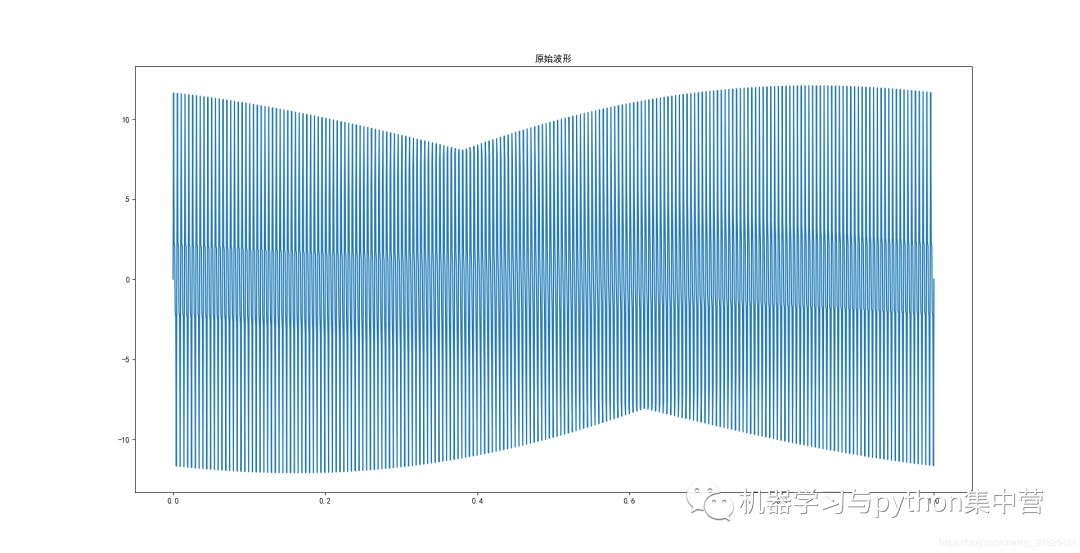由图可见，由于采样点太过密集，看起来不好看，我们只显示前面的50组数据，如下：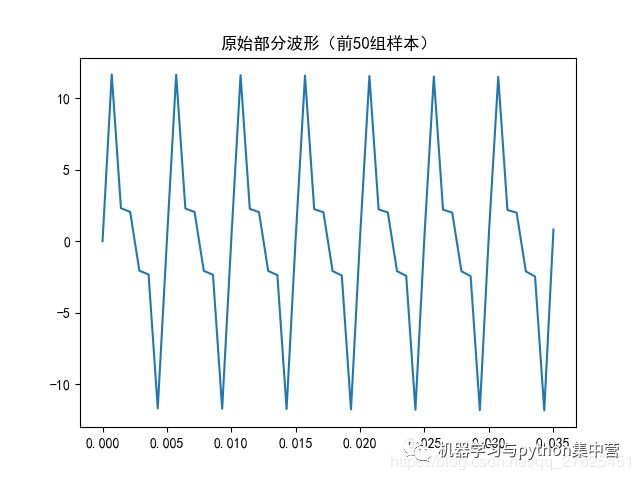2、快速傅里叶变换其实scipy和numpy一样，实现FFT非常简单，仅仅是一句话而已，函数接口如下：from scipy.fftpack import fft,ifftfrom numpy import fft,ifft其中fft表示快速傅里叶变换，ifft表示其逆变换。具体实现如下：fft_y=fft(y)                          #快速傅里叶变换print(len(fft_y))print(fft_y[0:5])'''运行结果如下：1400[-4.18864943e-12+0.j   9.66210986e-05-0.04305756j   3.86508070e-04-0.08611996j8.69732036e-04-0.12919206j    1.54641157e-03-0.17227871j]'''我们发现以下几个特点：（1）变换之后的结果数据长度和原始采样信号是一样的（2）每一个变换之后的值是一个复数，为a+bj的形式，那这个复数是什么意思呢？我们知道，复数a+bj在坐标系中表示为（a,b），故而复数具有模和角度，我们都知道快速傅里叶变换具有“振幅谱”“相位谱”，它其实就是通过对快速傅里叶变换得到的复数结果进一步求出来的，那这个直接变换后的结果是不是就是我需要的，当然是需要的，在FFT中，得到的结果是复数，（3）FFT得到的复数的模（即绝对值）就是对应的“振幅谱”，复数所对应的角度，就是所对应的“相位谱”，现在可以画图了。3、FFT的原始频谱N=1400x = np.arange(N)           # 频率个数abs_y=np.abs(fft_y)                # 取复数的绝对值，即复数的模(双边频谱)angle_y=np.angle(fft_y)              #取复数的角度plt.figure()plt.plot(x,abs_y)plt.title('双边振幅谱（未归一化）')plt.figure()plt.plot(x,angle_y)plt.title('双边相位谱（未归一化）')plt.show()显示结果如下：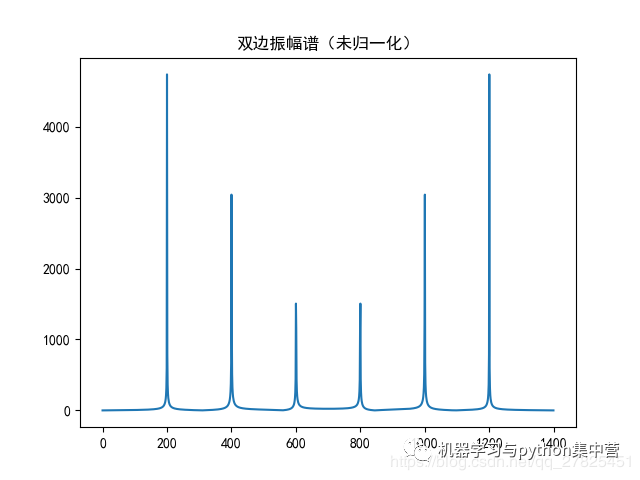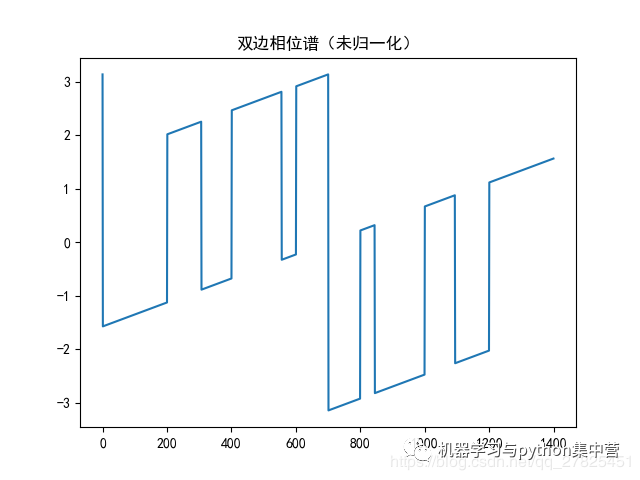注意：我们在此处仅仅考虑“振幅谱”，不再考虑相位谱。我们发现，振幅谱的纵坐标很大，而且具有对称性，这是怎么一回事呢？关键：关于振幅值很大的解释以及解决办法——归一化和取一半处理比如有一个信号如下：Y=A1+A2*cos(2πω2+φ2）+A3*cos(2πω3+φ3）+A4*cos(2πω4+φ4）经过FFT之后，得到的“振幅图”中，第一个峰值（频率位置）的模是A1的N倍，N为采样点，本例中为N=1400，此例中没有，因为信号没有常数项A1第二个峰值（频率位置）的模是A2的N/2倍，N为采样点，第三个峰值（频率位置）的模是A3的N/2倍，N为采样点，第四个峰值（频率位置）的模是A4的N/2倍，N为采样点，依次下去......考虑到数量级较大，一般进行归一化处理，既然第一个峰值是A1的N倍，那么将每一个振幅值都除以N即可FFT具有对称性，一般只需要用N的一半，前半部分即可。4、将振幅谱进行归一化和取半处理先进行归一化normalization_y=abs_y/N            #归一化处理（双边频谱）plt.figure()plt.plot(x,normalization_y,'g')plt.title('双边频谱(归一化)',fontsize=9,color='green')plt.show()结果为：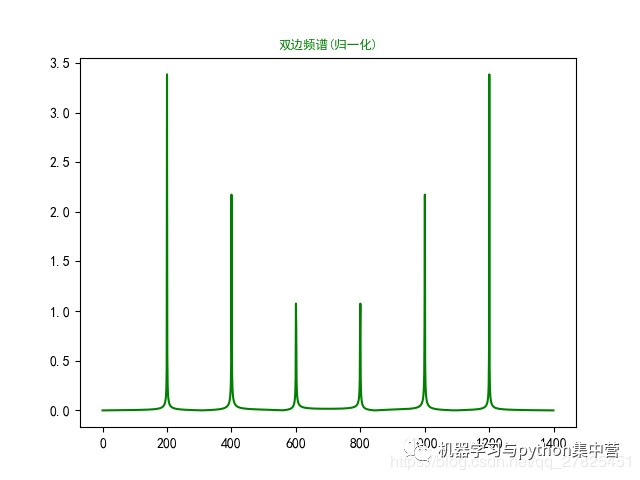现在我们发现，振幅谱的数量级不大了，变得合理了，接下来进行取半处理：half_x = x[range(int(N/2))]                                  #取一半区间normalization_half_y = normalization_y[range(int(N/2))]      #由于对称性，只取一半区间（单边频谱）plt.figure()plt.plot(half_x,normalization_half_y,'b')plt.title('单边频谱(归一化)',fontsize=9,color='blue')plt.show()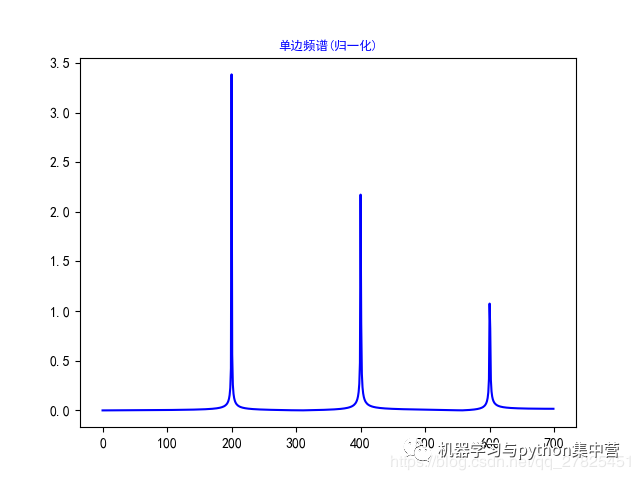这就是我们最终的结果，需要的“振幅谱”。三、完整代码import numpy as npfrom scipy.fftpack import fft,ifftimport matplotlib.pyplot as pltfrom matplotlib.pylab import mplmpl.rcParams['font.sans-serif'] = ['SimHei']   #显示中文mpl.rcParams['axes.unicode_minus']=False       #显示负号#采样点选择1400个，因为设置的信号频率分量最高为600赫兹，根据采样定理知采样频率要大于信号频率2倍，所以这里设置采样频率为1400赫兹（即一秒内有1400个采样点，一样意思的）x=np.linspace(0,1,1400)#设置需要采样的信号，频率分量有200，400和600y=7*np.sin(2*np.pi*200*x) + 5*np.sin(2*np.pi*400*x)+3*np.sin(2*np.pi*600*x)fft_y=fft(y)                          #快速傅里叶变换N=1400x = np.arange(N)             # 频率个数half_x = x[range(int(N/2))]  #取一半区间abs_y=np.abs(fft_y)                # 取复数的绝对值，即复数的模(双边频谱)angle_y=np.angle(fft_y)            #取复数的角度normalization_y=abs_y/N            #归一化处理（双边频谱）normalization_half_y = normalization_y[range(int(N/2))]      #由于对称性，只取一半区间（单边频谱）plt.subplot(231)plt.plot(x,y)plt.title('原始波形')plt.subplot(232)plt.plot(x,fft_y,'black')plt.title('双边振幅谱(未求振幅绝对值)',fontsize=9,color='black')plt.subplot(233)plt.plot(x,abs_y,'r')plt.title('双边振幅谱(未归一化)',fontsize=9,color='red')plt.subplot(234)plt.plot(x,angle_y,'violet')plt.title('双边相位谱(未归一化)',fontsize=9,color='violet')plt.subplot(235)plt.plot(x,normalization_y,'g')plt.title('双边振幅谱(归一化)',fontsize=9,color='green')plt.subplot(236)plt.plot(half_x,normalization_half_y,'blue')plt.title('单边振幅谱(归一化)',fontsize=9,color='blue')plt.show()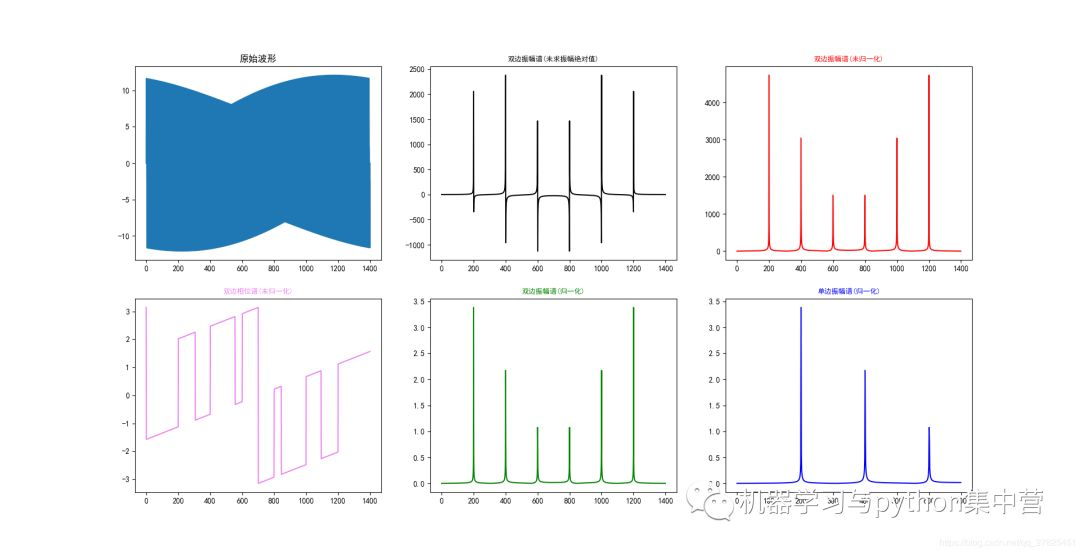疑问解答，关于归一化和取一半处理需看快速傅里叶变换在信号处理中的应用，具体为：傅里叶变换FT（Fourier Transform）是一种将信号从时域变换到频域的变换形式。它在声学、信号处理等领域有广泛的应用。计算机处理信号的要求是：在时域和频域都应该是离散的，而且都应该是有限长的。而傅里叶变换仅能处理连续信号，离散傅里叶变换DFT（Discrete Fourier Transform）就是应这种需要而诞生的。它是傅里叶变换在离散域的表示形式。但是一般来说，DFT的运算量是非常大的。在1965年首次提出快速傅里叶变换算法FFT（Fast Fourier Transform）之前，其应用领域一直难以拓展，是FFT的提出使DFT的实现变得接近实时。DFT的应用领域也得以迅速拓展。除了一些速度要求非常高的场合之外，FFT算法基本上可以满足工业应用的要求。由于数字信号处理的其它运算都可以由DFT来实现，因此FFT算法是数字信号处理的重要基石。傅立叶原理表明：任何连续测量的时序或信号，都可以表示为不同频率的正弦波信号的无限叠加。而根据该原理创立的傅立叶变换算法利用直接测量到的原始信号，以累加方式来计算该信号中不同正弦波信号的频率、振幅和相位。如图1所示，即为时域信号与不同频率的正弦波信号的关系。图中最右侧展示的是时域中的一个信号，这是一个近似于矩形的波，而图的正中间则是组成该信号的各个频率的正弦波。从图中我们可以看出，即使角度几乎为直角的正弦波，其实也是由众多的弧度圆滑的正弦波来组成的。在时域图像中，我们看到的只有一个矩形波，我们无从得知他是由这些正弦波组成。但当我们通过傅里叶变换将该矩形波转换到频域之后，我们能够很清楚的看到许多脉冲，其中频域图中的横轴为频率，纵轴为振幅。因此可以通过这个频域图像得知，时域中的矩形波是由这么多频率的正弦波叠加而成的。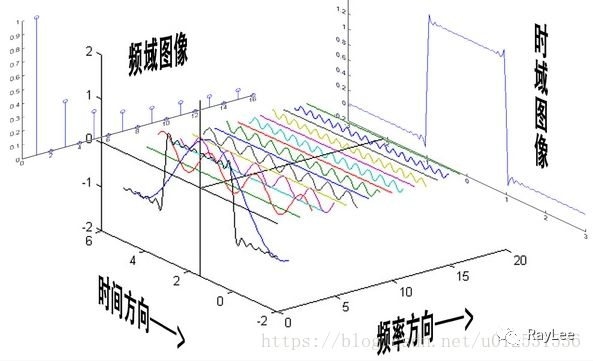这就是傅里叶变换的最基本最简单的应用，当然这是从数学的角度去看傅立叶变换。在信号分析过程中，傅里叶变换的作用就是将组成这个回波信号的所有输入源在频域中按照频率的大小来表示出来。傅里叶变换之后，信号的幅度谱可表示对应频率的能量，而相位谱可表示对应频率的相位特征。经过傅立叶变换可以在频率中很容易的找出杂乱信号中各频率分量的幅度谱和相位谱，然后根据需求，进行高通或者低通滤波处理，最终得到所需要频率域的回波。傅里叶变换在图像处理过程中也有非常重要的作用，设信号f是一个能量有限的模拟信号，则其傅里叶变换就表示信号f的频谱。从纯粹的数学意义上看，傅里叶变换是将一个函数转换为一系列周期函数来处理的。从物理效果看，傅里叶变换是将图像从空间域转换到频率域，其逆变换是将图像从频率域转换到空间域。换句话说，傅里叶变换的物理意义是将图像的灰度分布函数变换为图像的频率分布函数。傅里叶逆变换是将图像的频率分布函数变换为灰度分布函数。傅里叶频谱图上我们看到的明暗不一的亮点，其意义是指图像上某一点与邻域点差异的强弱，即梯度的大小，也即该点的频率的大小。一般来讲，梯度大则该点的亮度强，否则该点亮度弱。这样通过观察傅里叶变换后的频谱图，也叫功率图，我们就可以直观地看出图像的能量分布：如果频谱图中暗的点数更多，那么实际图像是比较柔和的，这是因为各点与邻域差异都不大，梯度相对较小；反之，如果频谱图中亮的点数多，那么实际图像一定是尖锐的、边界分明且边界两边像素差异较大的。以信号处理过程中的一个例子来详细说明FFT的效果：假设采样频率为Fs，信号频率为F，采样点数为N。那么FFT处理之后的结果就是一个点数为N点的复数。每一个点就对应着一个频率点，而每个点的模值，就是该频率值下的幅度特性。假设原始信号的峰值为A，那么在处理后除第一个点之外的其他点的模值就是A的N/2倍。而第一个点就是直流分量，它的模值就是直流分量的N倍。而每个点的相位呢，就是在该频率下的信号的相位。第一个点表示直流分量(即频率为0Hz)，而最后一个点N的再下一个点(实际上这个点是不存在的，这里是假设的第N+1个点，也可以看做是将第一个点分做两半分，另一半移到最后)则表示采样频率Fs，这中间被N-1个点平均分成N等份，每个点的频率依次增加。例如某点n所表示的频率为：Fn=(n-1)*Fs/N。由上面的公式可以看出，Fn 所能分辨到的最小频率为Fs/N，如果采样频率Fs为1024Hz，采样点数N为1024点，则最小分辨率可以精确到1Hz。1024Hz的采样率采样1024点，刚好是1秒，也就是说，采样1秒时间的信号并做FFT处理，则结果可以分析到1Hz；如果采样2秒时间的信号并做FFT处理，则结果可以精确到0.5Hz。假设现在我们有一个输入信号，该信号总共包含3种成分信号，其一是5V的直流分量；其二是频率为50Hz、相位为-60度、幅度为10V的交流信号；第三个成分信号是频率为100Hz、相位为90度、幅度为5V的交流信号。该输入信号用数学表达式表示如下：S=5+10*cos(2*pi*50*t-pi*60/180)+5*cos(2*pi*100*t+pi*90/180)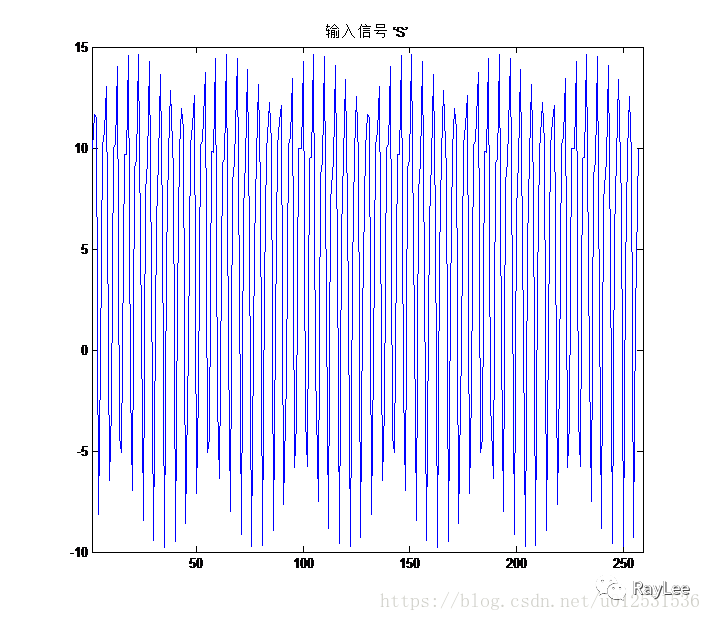图2即为S信号的图像表示。现在，我们以256Hz的采样率Fs对这个信号进行采样，采样点数N同样为256点。根据公式我们可以算出其频谱图中的频率精度为1Hz。因此对于输入信号频率包含0Hz、50Hz和100hz的复合信号，在其经过FFT处理之后，应该会在频谱图中出现3个峰值，而且频率分别为0Hz、50Hz和100Hz，处理结果如图3所示：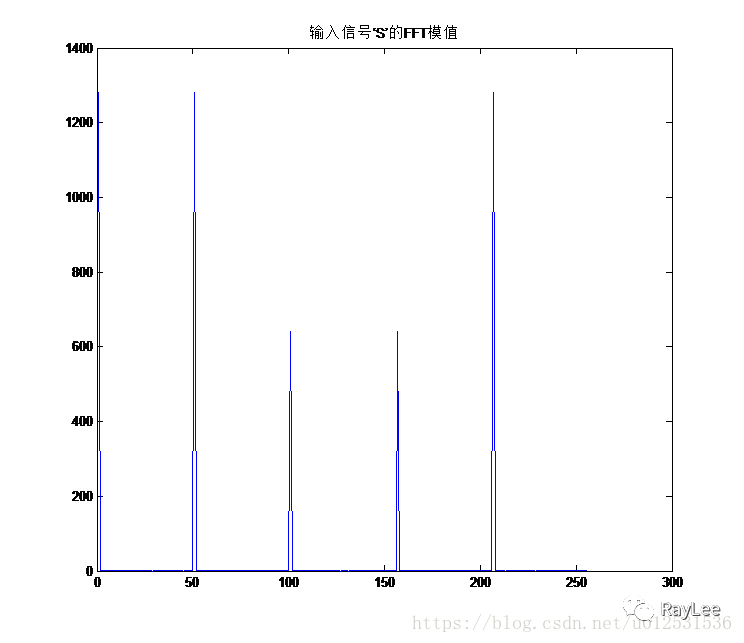结果正如我们所预料的，对输入信号’S’做FFT处理之后，图3中出现了5个峰值，这是因为对输入信号做256点的FFT处理之后并没有第257个频点信息，这也是前文中所提到的第一个点的模值是N倍的原因。因此，信号的 FFT结果具有一定的对称性。一般情况下，我们只使用前半部分的结果，即小于采样频率一半的结果。对于图像进行简单处理后，我们的前半部分的FFT结果如图4所示：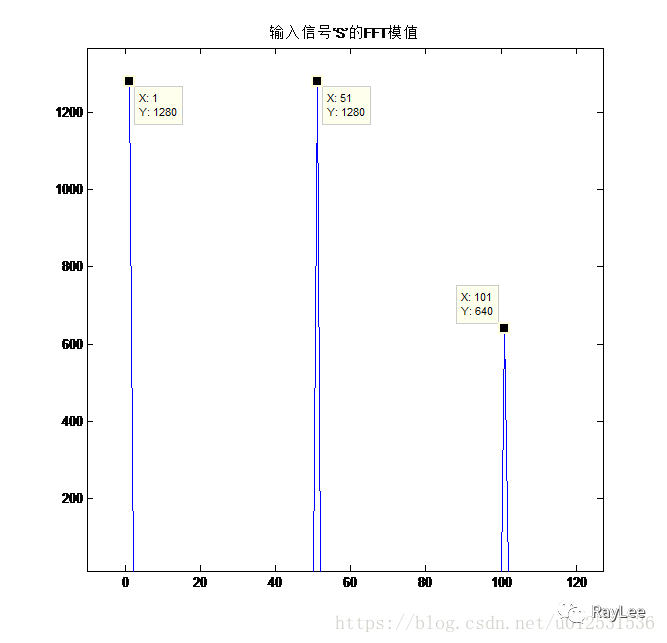从图4中可以看出，三个输入信号频点的幅值依次为1280、1280、640；其他频率所对应的幅值均为0。按照公式，可以计算直流分量（频率为0Hz）的幅值为：1280/N= 1280/256=5;频率为50Hz的交流信号的幅值为：1280/(N/2)= 1280/(256/2)=10;而75Hz的交流信号的幅值为640/(N/2)=640/(256/2)=5。这也正是我们输入信号中的三个分量的直流分量值，由此可见，从频谱分析出来的幅值是正确的。通过上面的例子可以看出，对于一个输入信号，假如我们不能确定该输入信号的频率组成，我们对其进行FFT处理之后，便可以很轻松的看出其频率分量，并且可以通过简单的计算来获知该信号的幅值信息等。另外，如果想要提高频率分辨率，我们根据计算公式首先想到的就是需要增加采样点数，但增加采样点数也就意味着计算量增加，这在工程应用中增加了工程难度。解决这个问题的方法有频率细分法，比较简单的方法是采样较短时间的信号，然后在后面补充一定数量的0，使其长度达到需要的点数（一般为2的幂次方的点数），然后再做FFT，就能在一定程度上提高频率分辨率。
展开全文• 提出一种将三角法测距与多频光栅相结合的方法，充分利用数字投影的RGB通道，将多频光栅与点阵同时投影在物体表面，综合控制受高度调制的相位信息的提取，最终获取物体的三维形貌。仿真结果显示，在高度跳变使高频...
• 二维离散傅里叶变换是将图像从空间域转至频域，在图像增强、图像去噪、图像边缘检测、图像特征提取、图像压缩等等应用中都起着极其重要的作用。理论基础是任意函数都可以表示成正弦函数的线性组合的形式。公式如下 ...
图像傅里叶变换
二维离散傅里叶变换是将图像从空间域转至频域，在图像增强、图像去噪、图像边缘检测、图像特征提取、图像压缩等等应用中都起着极其重要的作用。理论基础是任意函数都可以表示成正弦函数的线性组合的形式。公式如下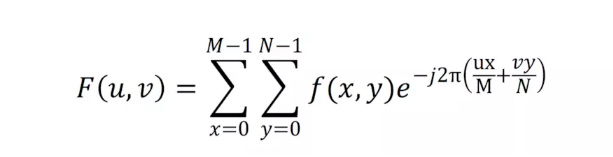逆变换公式如下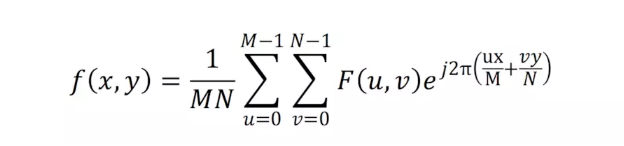令 R(u,v) 和 I(u,c) 分别表示 F(u,v) 的实部和虚部。
幅度谱为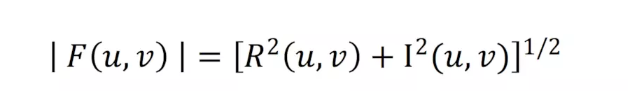相位谱为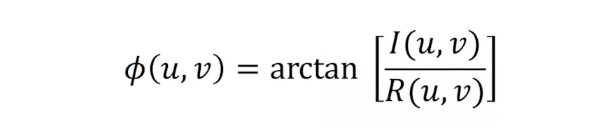指数表示功率谱为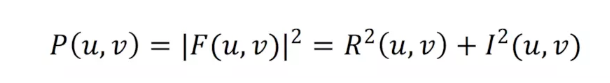示例演示
首先我们演示下，从一幅图像得到其的幅度谱和相位谱，然后再根据幅度谱和相位谱还原图像。代码如下。
void MainWindow::dftTransform(cv::Mat &image)
{
image.convertTo(image, CV_32F);
std::vector<cv::Mat> channels;
split(image, channels);  //分离图像的RGB通道，
cv::Mat image_B = channels; //OpenCV:BGR
//expand input image to optimal size
int m1 = cv::getOptimalDFTSize(image_B.rows);  //选取最适合做fft的宽和高
int n1 = cv::getOptimalDFTSize(image_B.cols);
//填充0
cv::copyMakeBorder(image_B, padded, 0, m1 - image_B.rows, 0, n1 - image_B.cols, cv::BORDER_CONSTANT, cv::Scalar::all(0));
cv::Mat complexI;
cv::merge(planes, 2, complexI);  //planes, planes是实部和虚部

cv::dft(complexI, complexI, cv::DFT_SCALE | cv::DFT_COMPLEX_OUTPUT);
cv::split(complexI, planes);

//定义幅度谱和相位谱
cv::Mat ph, mag, idft;
cv::phase(planes, planes, ph);
cv::magnitude(planes, planes, mag);  //由实部planes和虚部planes得到幅度谱mag和相位谱ph

cv::imshow("phase", ph);
cv::imshow("magnitude", mag);
/*
如果需要对实部planes和虚部planes，或者幅度谱mag和相位谱ph进行操作，在这里进行更改
*/

cv::polarToCart(mag, ph, planes, planes);  //由幅度谱mag和相位谱ph恢复实部planes和虚部planes
cv::merge(planes, 2, idft);
cv::dft(idft, idft, cv::DFT_INVERSE | cv::DFT_REAL_OUTPUT);
image_B = idft(cv::Rect(0, 0, image.cols & -2, image.rows & -2));
image_B.copyTo(channels);
merge(channels, image);
image.convertTo(image, CV_8U);
cv::imshow("idft", image);
}

运行结果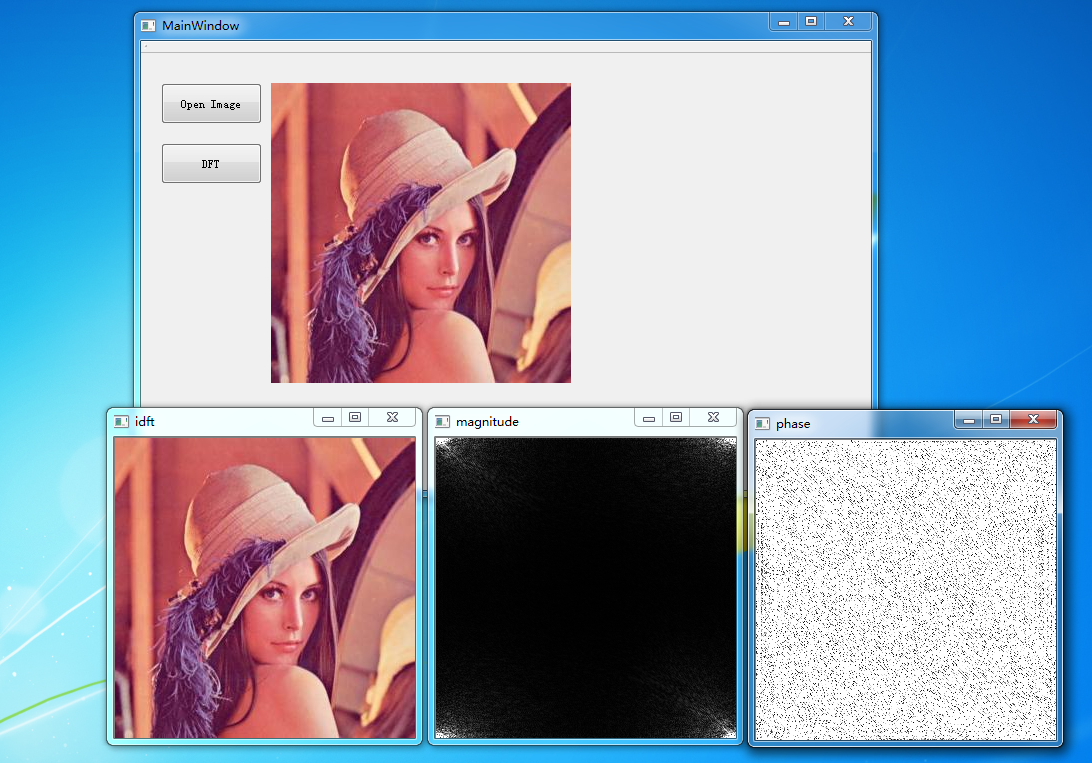展开全文图像处理
• 在信号处理领域，存在诸多变换，比如标题中的五个变换。本文将对这五个变换进行介绍和比较。在开始之前，我们需要先理清什么是...通常傅里叶变换只适合处理平稳信号，对于非平稳信号，由于频率特性会随时间变化，为了
在信号处理领域，存在诸多变换，比如标题中的五个变换。本文将对这五个变换进行介绍和比较。在开始之前，我们需要先理清什么是平稳信号，什么是非平稳信号。
我们知道，自然界中几乎所有信号都是非平稳信号，比如我们的语音信号就是典型的非平稳信号。那么何谓平稳信号和非平稳信号呢？一个通俗的理解即，平稳信号在不同时间得到的采样值的统计特性(比如期望、方差等)是相同的，非平稳信号则与之相反，其特性会随时间变化。在信号处理中，这个特性通常指频率。
通常傅里叶变换只适合处理平稳信号，对于非平稳信号，由于频率特性会随时间变化，为了捕获这一时变特性，我们需要对信号进行时频分析，就包括短时傅里叶变换、小波变换、希尔伯特变换、希尔伯特黄变换这几种变换。以下逐一进行分析介绍。
傅里叶变换(Fourier Transform, FT)

首先考虑一个连续信号$f(t)$的傅里叶变换和它的反变换，如下
$F(\omega)=F[f(t)]=\int_{-\infty}^{+\infty}f(t)e^{-j\omega t}dt$
$f(t)=F^{-1}[F(\omega)]=\frac{1}{2\pi}\int_{-\infty}^{+\infty}F(\omega)e^{j\omega t}d\omega$
在实际应用中，计算机只能处理离散信号，所以对连续信号$x(t)$进行时域采样，得到一组离散样本$x(n)$，对它进行傅里叶变换得到
$X(\omega)=\sum\limits_{n=-\infty}^\infty x(n)e^{-j\omega n}$
上式即为离散时间傅里叶变换(DTFT)，由于变换后得到的频域值仍然是连续的，我们继续对频域进行采样，得到
$X(k)=\sum\limits_{n=0}^{N-1}x(n)e^{-j\frac{2πkn}{N}}$
上式就是离散傅里叶变换(DFT)，当前计算机中常用的快速傅里叶变换(FFT)，就是DFT的快速算法。如下即为信号$x(t)=cos(200\pi t)+0.5cos(400\pi t)$的采样信号和经过FFT得到的幅度谱，其中采样频率$f_s=500Hz$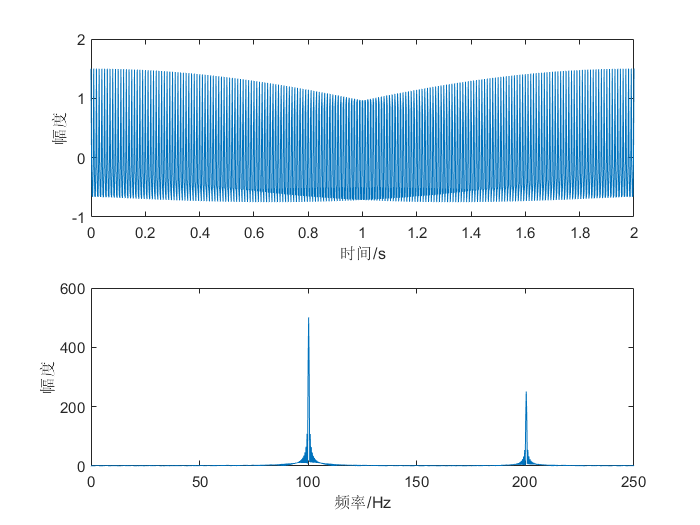可以看到，该信号由两个频率分别为100Hz和200Hz的分量构成。利用频谱分析，我们可以发现一些时域中看不到的信息，比如信号的频率分量组成、信号能量在频域上的分布等。
然而，傅里叶变换是一种全局的变换，时域信号经过傅里叶变换后，就变成了频域信号，从频域是无法看到时域信息的，我们可以从上节中的傅里叶变换和反变换公式进行解释，进行正变换时，积分区间为整个时域，所以变换结果将不包含时域信息，反变换同理。
如果信号的频率特性在任何时间都不发生改变(即该信号是平稳信号)的话，使用傅里叶变换是没有问题的，然而如果该信号是非平稳信号，这时候时域信息就相当重要了。举个栗子，以下分别是0100Hz线性递增扫频信号和1000Hz线性递减扫频信号的幅度谱，这两个信号都是非平稳信号，可以看到它们的幅度谱是相同的，很显然我们无法知道每一个频率分量出现的时间，即无法知道扫频信号的模式。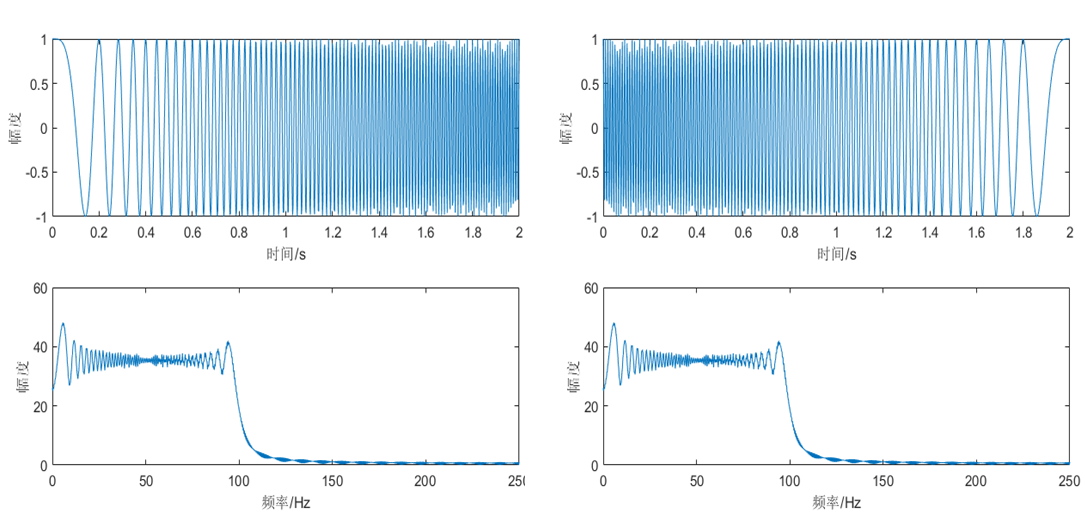上述例子可能比较简单，可以直接从时域信号看出扫频模式，但是对于一些复杂的信号和它的幅度谱，比如以下的两路扫频信号叠加后的信号和它的幅度谱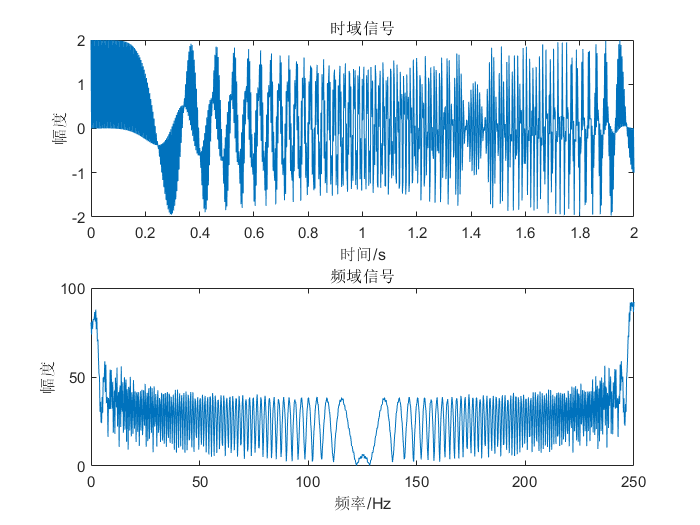仅仅通过时域信号或者幅度谱，我们是很难分析这段非平稳信号的特征的。下面我们将引入一个时频分析( Time-Frequency Analysis)方法——短时傅里叶变换(STFT)。
短时傅里叶变换(Short-Time Fourier Transform, STFT)

短时傅里叶变换定义为
$X(n,\omega)=\sum_{m=-\infty}^\infty x(m)w(n-m)e^{-j\omega m}$
其中$x(m)$为输入信号，$w(m)$是窗函数，它在时间上反转并且有n个样本的偏移量。$X(n,\omega)$是时间$n$和频率$\omega$的二维函数，它将信号的时域和频域联系起来，我们可以据此对信号进行时频分析，比如$S(n,\omega)=|X(n,\omega)|^2$就是语音信号所谓的语谱图(Spectrogram)。
画出上节中两路扫频信号叠加后的信号的语谱图，如下图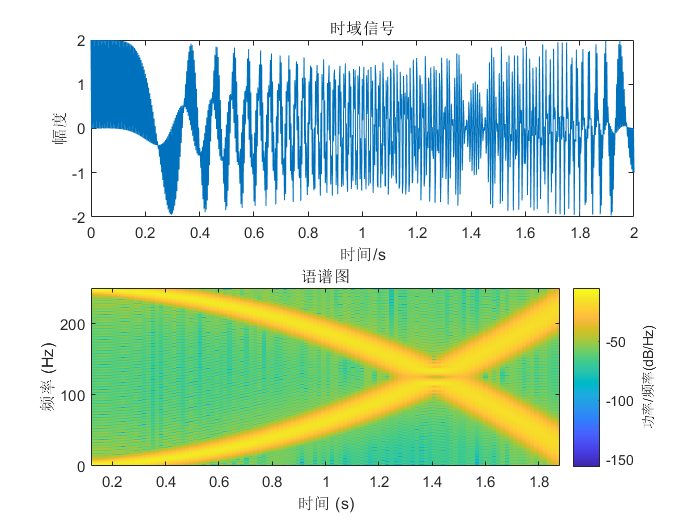可见该信号是由一个0 ~ 250Hz二次递增的扫频信号和一个250 ~ 0Hz二次递减的扫频信号的叠加。通过STFT，我们可以很容易地得出非平稳信号的时变特性。
继续对STFT进行分析。
计算语谱$S(n,\omega)$时采用不同窗长度，可以得到两种语谱图，即窄带和宽带语谱图。长时窗（至少两个基音周期）常被用于计算窄带语谱图，短窗则用于计算宽带语谱图。窄带语谱图具有较高的频率分辨率和较低的时间分辨率，良好的频率分辨率可以让语音的每个谐波分量更容易被辨别，在语谱图上显示为水平条纹。相反宽带语谱图具有较高的时间分辨率和较低的频率分辨率，低频率分辨率只能得到谱包络，良好的时间分辨率适合用于分析和检验英语语音的发音。
如下图所示，分别为一段语音的帧长为128和512的语谱图。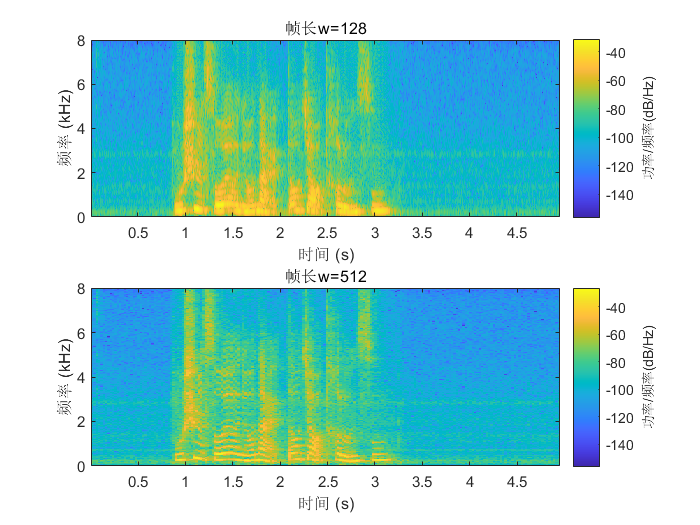可见，对于帧长固定的短时傅里叶变换，在全局范围内的时间分辨率和频率分辨率是固定的。如果我们想要在低频区域具有高频率分辨率，在高频区域具有高时间分辨率，显然STFT是不能满足要求的。我们继续引入另一种时频分析方法——小波变换。
小波变换(Wavelet Transform, WT)

对于任意能量有限信号$f(t)$，其连续小波变换(CWT)定义为
$W_f(a,b)=\frac{1}{\sqrt a}\int_{-\infty}^{+\infty}f(t)\psi^*(\frac{t-b}{a})dt$
其中$\psi(t)$是母小波或者基本小波，它满足$\psi(±\infty)=0$，$\psi(0)=0$，$\int_{-\infty}^{+\infty}\psi(t)dt=0$，前两个条件表明$\psi(t)$在时域上是一个有限长的函数，最后一个条件则表明$\psi(t)$必须时正时负地波动，否则它的积分结果不会为零，因此它在频域上也是有限的。所以不同于傅里叶变换的基函数是一个无限长的正弦波，小波变换的基函数是一个经过衰减处理的有限长小波，小波基函数在时域和频域上都是局部化的，如下图分别为傅里叶变换和小波变换的基函数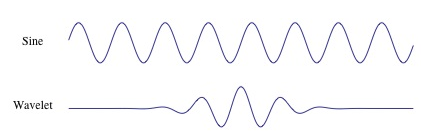将$\psi(t)$进行伸缩和平移得到一族函数$\psi_{a,b}(t)$，称为分析小波，这就是小波变换的基函数族，其中$a$为伸缩参数，当$a>1$时，沿时间轴方向拉伸，因子$1/\sqrt a$是为了保持伸缩之后能量不变；$b$为平移参数。
使用MATLAB的小波变换工具箱画出上节两路扫频信号叠加信号的小波变换结果，如下图，其中纵轴(频率轴)是对数轴。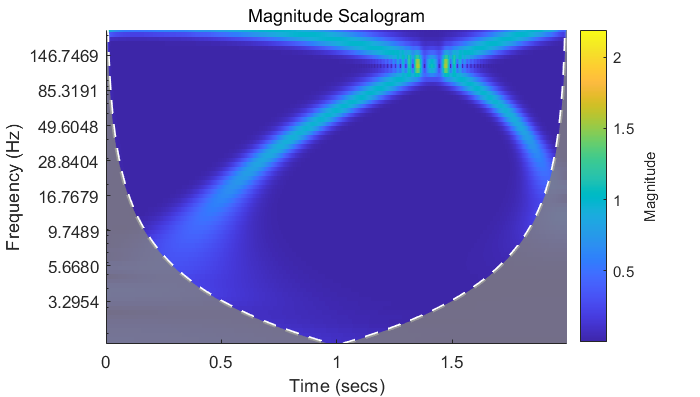可见在低频区域的变换结果具有较高的频率分辨率(频率轴是对数轴，在低频区域跨度较小)，在高频区域具有较高的时间分辨率。
一些总结
我们对上述方法进行总结，可以发现上述方法的区别都可以归结为选取的时频窗尺寸不同。画出时域信号、频域信号、使用STFT得到的时频域信号、使用小波变换得到的时频域信号的时频窗，如下图，每一个小方块表示一个时频窗，沿时间方向的边长表示时间分辨率，沿频率方向的边长表示频率分辨率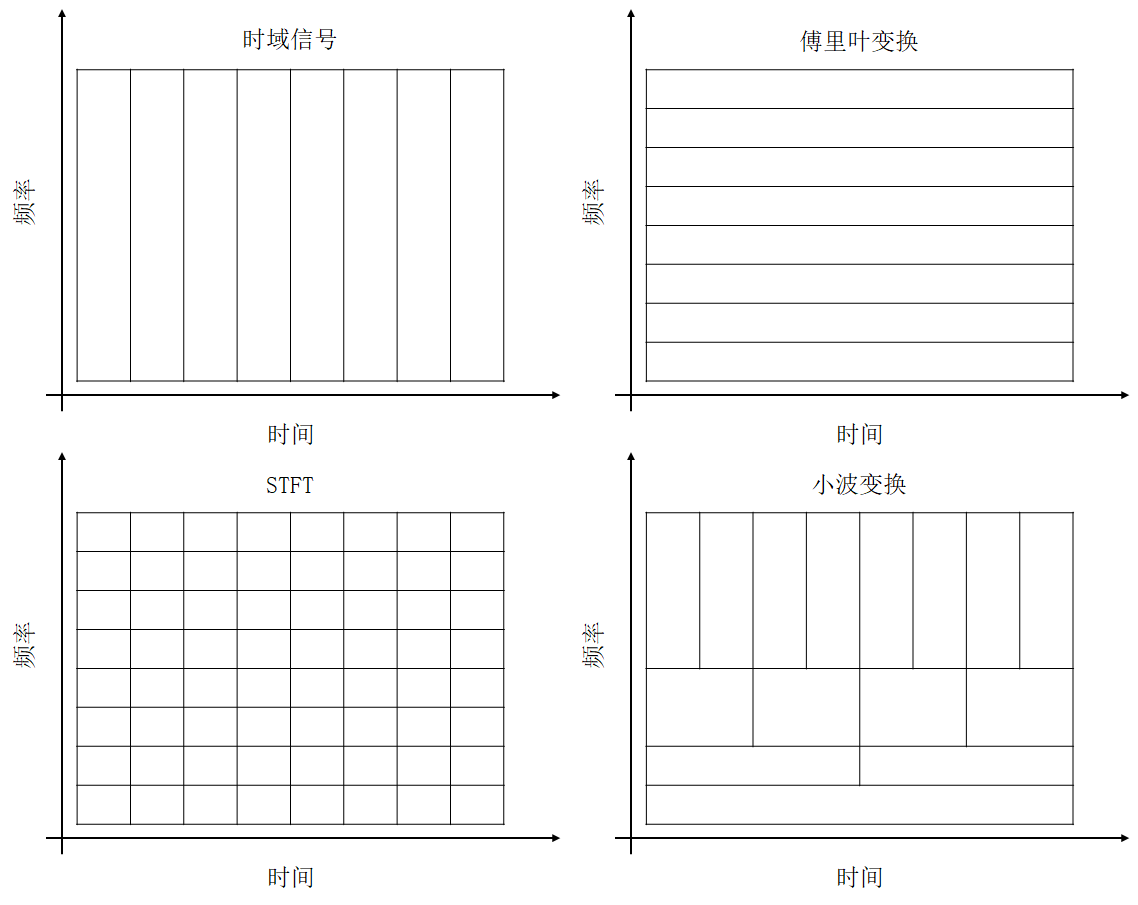由上图可以知道：

对于时域信号，它可以有很高的时间分辨率，然而其频率分辨率为零。

经过傅里叶变换得到的频域信号可以实现很高的频率分辨率，然而其时间分辨率为零。

对于短时傅里叶变换(STFT)，它在时域和频域都有一定的分辨率，并且在全局范围内STFT的时频分辨率都是一样的。但是由于Heisenberg不确定原理(也就是量子力学中的测不准原理)的制约，每一个时频窗的面积都是固定的，即时间分辨率和频率分辨率成反比，所以这两个分辨率不能同时很高。

小波变换在不同时间和频率上具有不同尺寸的时频窗，可以在低频区域实现较高的频率分辨率，然而其仍然受到Heisenberg不确定原理的限制，时间分辨率和频率分辨率不能两全其美。同时小波变换的时频窗并非完全是自适应的，它还需要人为地选择基函数。

上述的方法都会受到Heisenberg不确定原理的限制，而且并不是完全自适应的方法。接下来介绍一种不受Heisenberg不确定原理限制、同时还有更好的自适应性的时频分析方法——希尔伯特黄变换
希尔伯特变换(Hilbert Transform, HT)

在介绍希尔伯特黄变换之前，我们先介绍一下希尔伯特变换。
希尔伯特变换也是傅里叶变换的一种扩展，它常常用于通信系统中的调制解调，当然它也可以用于信号的时频分析。其计算方法为

计算输入信号的FFT，保存为向量F

创建一个向量h，其中
$h(i)=\begin{cases}1&,i=1,\frac{n}{2}+1 \\2&,i=2,3,\cdots,\frac{n}{2}\\0&,i=\frac{n}{2}+2,\cdots,n\end{cases}$

计算F与h的内积

计算上步得到的序列的iFFT

稍微介绍下它在通信系统中的应用，利用MATLAB中的hilbert函数对一个扫频信号进行希尔伯特变换，得到的结果是一个解析信号，在同一坐标系画出该解析信号的实数部分和虚数部分，如下图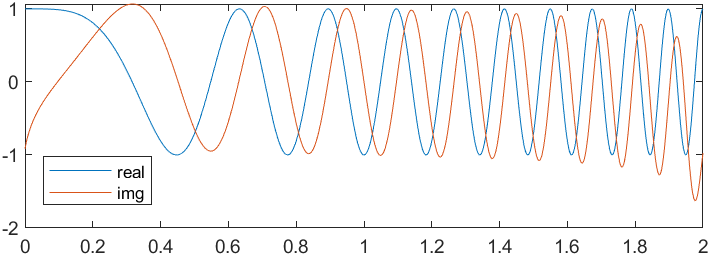可见虚数部分较实数部分滞后$\pi / 2$，我们可以利用这个性质消掉负频率。使用MATLAB中的fft函数对一个信号进行傅里叶变换，结果中的负频率保存在下半轴。如下是一个扫频信号的幅度谱以及使用希尔伯特变换得到的解析信号的幅度谱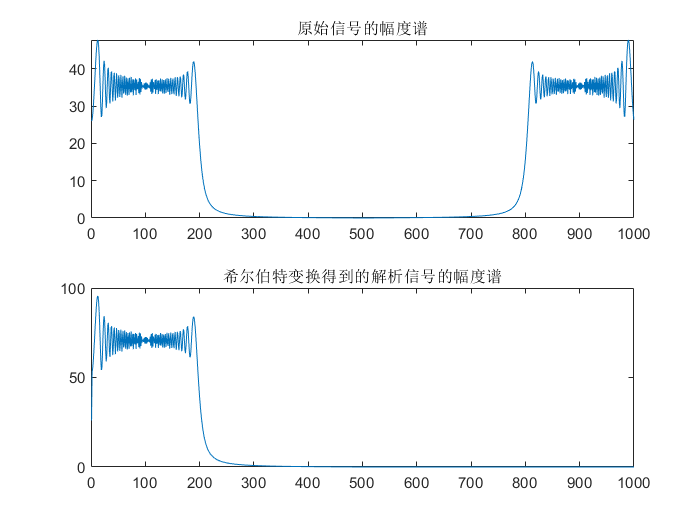由上图可见，解析信号的幅度谱没有负频率，并且各个频率分量的幅度是原来实信号的两倍。
通过希尔伯特变换可以得到没有负频率的解析信号，基于此可以实现信号的单边带调制，从而节省带宽和降低发射功率。感兴趣可以继续查看使用希尔伯特变换进行单边带幅度调制
继续探讨基于希尔伯特变换的时频分析。在时频分析领域，希尔伯特变换主要用于瞬时频率估计。
由上述分析可知使用希尔伯特变换可以得到原始信号的解析信号，假设解析信号为$z(t)$
$z(t)=c(t)+jy(t)=ae^{j\theta(t)}$
其中$a(t)=\sqrt{c(t)^2+y(t)^2}$，表示瞬时幅值，$\theta(t)=arctan\frac{y(t)}{c(t)}$，表示瞬时相位，$\omega=\frac{d\theta(t)}{dt}$，表示瞬时频率。由瞬时幅值和瞬时频率可将信号表示为
$x(t)=a(t)e^{j\int\omega(t)dt}$
若使用$|a(t)|^2$表示瞬时能量，则可在时间-频率面上画出信号的瞬时能量分布，这个分布谱图就是Hilbert谱，记为$H(\omega,t)$。如下为一个0~100Hz扫频信号的时域信号和它的Hilbert谱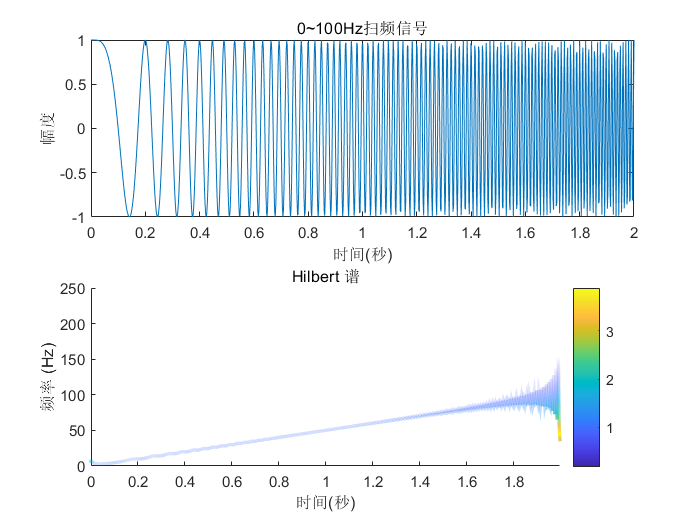上图中的信号为单频率成分信号，即同一时刻只有一个频率分量的信号，我们可以由Hilbert谱很好地观察出信号的时频特征，且有很高的的时间分辨率，但是信号边界处的误差往往较大。
如果是对一个多频率成分信号(同一时刻有多个频率分量的信号)进行希尔伯特变换，结果会怎样呢？如下为两路扫频信号叠加信号的Hilbert谱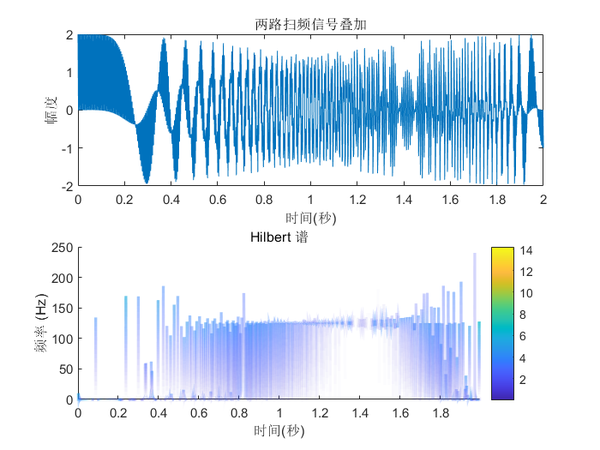从上图中的Hilbert谱我们并不能概括出该信号的时频特征，所以对多频率成分信号不能直接进行Hilbert变换，我们还需要对其进行进一步处理，将原始信号分解成单频率信号的叠加，这就要用到希尔伯特黄变换中的EMD分解。
希尔伯特黄变换(Hilbert-Huang Transform, HHT)

相比于HT，HHT就多了一个经验模态分解(Empirical Mode Decomposition, EMD)，EMD就是把复杂信号分解成从高频到低频的若干个固有模态函数(Intrinsic Mode Function, IMF)，IMF需要满足两个条件：

信号极值点的数量与零点数相等或相差为1
信号的由极大值定义的上包络和由极小值定义 的下包络的局部均值为0(即包络上下对称)

简单的理解就是，EMD是依次提取信号在每个局部的最高频分量的过程，所以每个IMF实际上是一个单频率分量信号，这样我们就可以对每个IMF分量进行Hilbert变换，从而得到每个分量的Hilbert谱。如下是对两个扫频信号叠加后的信号进行EMD分解得到的IMF分量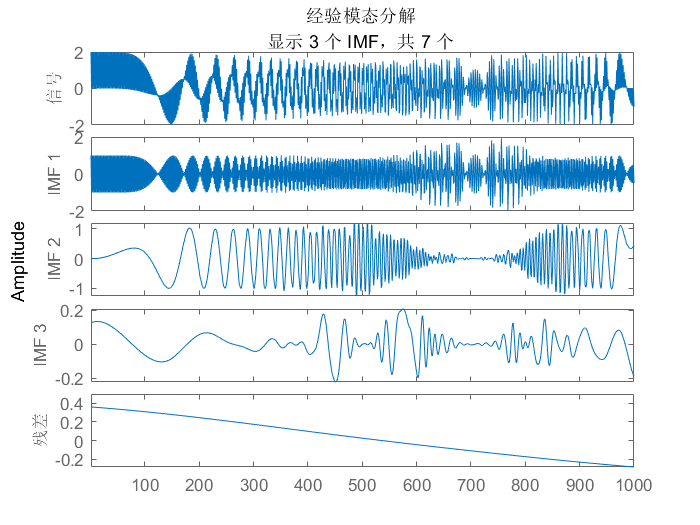对上述的IMF分量进行Hilbert变换，求得Hilbert谱，如下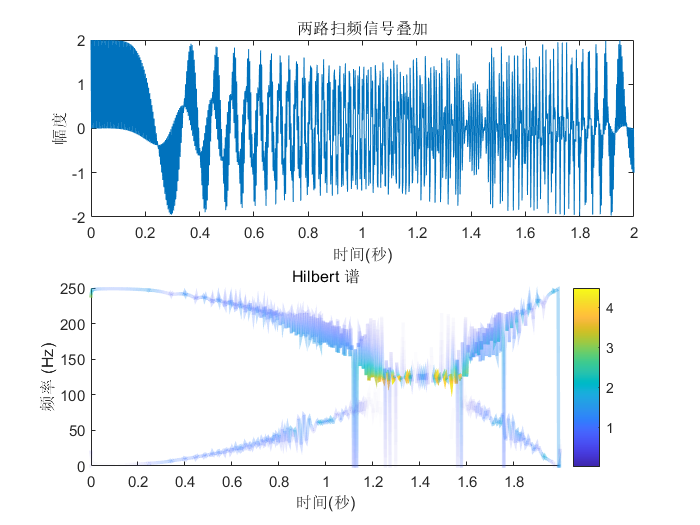从上图我们大致可以看出信号的时频特性：该信号是一个0 ~ 250Hz二次递增的扫频信号和一个250 ~ 0Hz二次递减的扫频信号的叠加。
当然HHT并不是完美的，目前对于它的关键步骤EMD分解的研究尚不完善，缺乏一些理论基础。从上图我们也可以看到，HHT在低频区域可能会出现一些不存在的频率分量。
参考

https://blog.csdn.net/Forlogen/article/details/88535027
https://www.sohu.com/a/246972969_607269
唐晓初. 小波分析及其应用[M]. 重庆大学出版社, 2006.
Marple L . Computing the discrete-time “analytic” signal via FFT[J]. IEEE Transactions on Signal Processing, 1999.
罗利春. 用希尔伯特变换构造解析信号进行时频分析[J]. 航天电子对抗, 2003(03):27-30.
Qian S , Chen D . Joint time-frequency analysis[J]. IEEE Signal Processing Magazine, 1999, 16(2): P.52-67.


展开全文信号处理 数字信号处理 matlab 小波分析
• 目的：读取图像 A(lena.tiff)和B(rice.tif)，显示这两幅图像，对图像作傅立叶变换，显示图像的傅里叶幅度谱和相位谱。做傅立叶逆变换，显示重建图像。图像的频率是表征图像中灰度变化剧烈程度的指标，是灰度在平面...
• 提出一种基于快速傅里叶变换(FFT)提取相位的方法,分析自混合干涉信号,不增加系统复杂度就能将激光自混合(SMLD)微位移测量精度提高到纳米量级。在激光自混合微位移测量原理的基础上,介绍了FFT提取相位信号处理方法的...
• 原标题：让你永远忘不了的傅里叶变换解析作者：刘遥行来自：直观详解】让你永远忘不了的傅里叶变换解析/使用联想链条和几何直观，辅以从实际需求衍生概念的思考模式，详解 什么是傅立叶变换，为什么要做傅立叶变换等...
• 争取从零开始 一遍搞懂。 本章：【由于字数限制开新文章继续】复指数信号、傅里叶级数的系数推导、三角函数正交性、离散傅里叶变换相位补偿、z变换表、逆变换表、常见序列及其作用信号处理 算法 matlab 线性代数
• 一、前言我想认真写好快速傅里叶变换（Fast Fourier Transform，FFT），所以这篇文章会由浅到细，由窄到宽的讲解，但是傅里叶变换对于寻常人并不是很容易理解的，所以对于基础不牢的人我会通过前言普及一下相关知识...
• 傅里叶变换是一种常见的频域变换，它可以实现图像增强、图像去噪、边缘检测、特征提取、图像压缩和加密等。 一：理论基础 法国数学家指出：任何周期函数都可以表示为不同频率的正弦函数和的形式。它有三个关键的要素...
• 通俗理解傅里叶变换，先看这篇文章傅里叶变换的通俗理解！ 　接下来便是使用python进行傅里叶FFT-频谱分析： 一、一些关键概念的引入 1、离散傅里叶变换（DFT） 离散傅里叶变换(discrete Fourier transform) ...
• ## 图像傅里叶变换

千次阅读 2018-04-27 16:14:26
1. 图像的傅里叶变换傅里叶变换可以看成是时域和频域的转换。一维图像傅里叶变换公式（空间域-&gt;频域）：一维傅里叶变换变换公式（频域-&gt;空间域）：M×N图像的二维离散傅里叶变换：M×N图像的傅里叶...频谱图分析
• 傅里叶变换，是把信号从时间域变换到频率域，进而研究信号的频谱结构和变化规律。上一篇文章中，我们讨论了Python、正弦波、复数。本篇文章中，我们继续用Python中的numpy+matplotlab的方式来实现复数正弦波和离散...Python 人工智能 图像识别 信号处理人工智能 opencv
• 提出一种基于分数傅里叶变换和随机相位编码的光学加密数字水印技术,可成为一种信息隐藏及保护的有效方案。该数字水印技术对于噪音叠加和常见的图像处理操作具有较强的稳健性。该技术根据光学级联分数傅里叶变换系统,...
• 一、基于傅里叶变换的图像校正 由于拍照时，图像被旋转，为方便观看或使用，需要对旋转图像进行校正。 旋转图像矫正流程 思路借鉴 http://johnhany.net/2013/11/dft-based-text-rotation-correction/ 获取图像...
• 3.7 图像变换-傅里叶变换 学习目标 理解傅里叶变换 知道傅里叶变换的相关概念 知道傅里叶变换的分类 知道怎么在图像中进行傅里叶变换 了解傅里叶变换在图像应用中的意义 知道在OPenCV...opencv 计算机视觉 机器学习 python 神经网络...